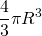# Solution assignment 08 Area and volume

### Assignment 8

Calculate the volume of the sphere with center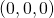and radius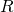.

### Solution

First we look at the circle in the first quadrant and its graph can be represented by the function: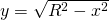We revolve this graph around the-axis and thus get the volume of the half sphere.
So we get: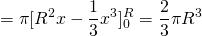The volume of the whole sphere is: